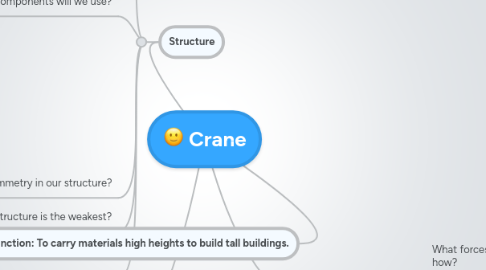# Crane

Get Started. It's FreeCrane## 1. Form

### 1.1. What kind of structure are we going to make solid, shell, frame or combination?

1.1.1. If we do combination what parts will be frame, which will be solid and which will be shell?

### 1.2. What materials will we use?

1.2.1. Will mixing the materials together make them stronger?

## 2. Forces

### 2.1. What forces might impact the structure? And how?

2.1.1. How much impact can it take?

### 2.2. What live loads could it have?

2.2.1. How much live loads can it carry?

2.2.1.1. How much weight can it carry?

2.2.1.1.1. Could the weight of the live load crush it?

### 2.3. What dynamic loads could it have?

2.3.1. How much impact can it handle?

## 3. Structure

### 3.1. Where is the centre of gravity?

3.1.1. How can we lower the centre of gravity?

### 3.2. What structural components will we use?

3.2.1. Which structural component will support the structure the best?

3.2.2. How can we use a tie or strut?

3.2.3. How can we use a girder?

3.2.4. How can we use an I-beam?

3.2.5. How can we use a column?

3.2.6. How can we use a gusset?

3.2.7. How can we use a truss?

3.2.7.1. What kind of truss would we use? A pratt truss, a warren truss, or a howe truss?

3.2.8. How can we use lamination?

3.2.9. How can we use an arch?

### 3.3. Can we use symmetry in our structure?

3.3.1. Can we balance out the weight?

### 3.4. What point on the structure is the weakest?

3.4.1. How can we make it stronger?